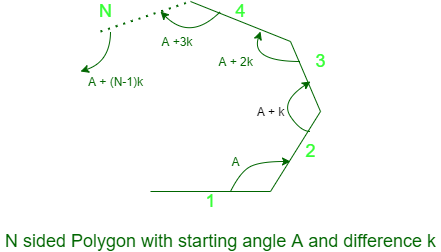GeeksforGeeks App
Open AppBrowser
Continue

# Nth angle of a Polygon whose initial angle and per angle increment is given

Given four integers N, A, K, n where N represents the number of sides the polygon, A represents the initial angle of the polygon, K represents the per angle increase, the task is to find the nth angle of the polygon having N sides. If it is not possible then print -1.Examples:

Input: N = 3, A = 30, K = 30, n = 3
Output: 90
Explanation:
The 3rd angle of the polygon having three sides is 90 degree as the all angles are 30, 60, 90.

Input: N = 4, A = 40, K = 30, n = 3
Output: -1
Explanation:
It is not possible to create that polygon whose initial angle is 40 and no. of sides are 4.

Approach: The idea is to observe that the angles of the polygon are increasing in the terms of Arithmetic Progression by a difference of K degree.

• Find out the angular sum of the N sided polygon and the sum of the angles of the given polygon.
• Check if both the values are equal or not. If yes, then the nth angle is possible hence find the nth angle.
• Otherwise, print -1.

Below is the implementation of the above approach:

## C++

 `// C++ program for the above approach``#include ``using` `namespace` `std;` `// Function to check if the angle``// is possible or not``bool` `possible(``int` `N, ``int` `a, ``int` `b, ``int` `n)``{``    ``// Angular sum of a N-sided polygon``    ``int` `sum_of_angle = 180 * (N - 2);` `    ``// Angular sum of N-sided given polygon``    ``int` `Total_angle = (N * ((2 * a) + (N - 1) * b)) / 2;` `    ``// Check if both sum are equal``    ``if` `(sum_of_angle != Total_angle)``        ``return` `false``;``    ``else``        ``return` `true``;``}` `// Function to find the nth angle``int` `nth_angle(``int` `N, ``int` `a,``              ``int` `b, ``int` `n)``{``    ``int` `nth = 0;` `    ``// Calculate nth angle``    ``nth = a + (n - 1) * b;` `    ``// Return the nth angle``    ``return` `nth;``}` `// Driver Code``int` `main()``{` `    ``int` `N = 3, a = 30, b = 30, n = 3;` `    ``// Checks the possibility of the``    ``// polygon exist or not``    ``if` `(possible(N, a, b, n))` `        ``// Print nth angle``        ``// of the polygon``        ``cout << nth_angle(N, a, b, n);``    ``else``        ``cout << ``"Not Possible"``;` `    ``return` `0;``}`

## Java

 `// Java program for the above approach``class` `GFG{` `// Function to check if the angle``// is possible or not``static` `boolean` `possible(``int` `N, ``int` `a,``                        ``int` `b, ``int` `n)``{``    ` `    ``// Angular sum of a N-sided polygon``    ``int` `sum_of_angle = ``180` `* (N - ``2``);` `    ``// Angular sum of N-sided given polygon``    ``int` `Total_angle = (N * ((``2` `* a) +``                      ``(N - ``1``) * b)) / ``2``;` `    ``// Check if both sum are equal``    ``if` `(sum_of_angle != Total_angle)``        ``return` `false``;``    ``else``        ``return` `true``;``}` `// Function to find the nth angle``static` `int` `nth_angle(``int` `N, ``int` `a,``                     ``int` `b, ``int` `n)``{``    ``int` `nth = ``0``;` `    ``// Calculate nth angle``    ``nth = a + (n - ``1``) * b;` `    ``// Return the nth angle``    ``return` `nth;``}` `// Driver Code``public` `static` `void` `main(String[] args)``{``    ``int` `N = ``3``, a = ``30``, b = ``30``, n = ``3``;` `    ``// Checks the possibility of the``    ``// polygon exist or not``    ``if` `(possible(N, a, b, n))``        ` `        ``// Print nth angle``        ``// of the polygon``        ``System.out.print(nth_angle(N, a, b, n));``    ``else``        ``System.out.print(``"Not Possible"``);``}``}` `// This code is contributed by amal kumar choubey`

## Python3

 `# Python3 program for the above approach` `# Function to check if the angle``# is possible or not``def` `possible(N, a, b, n):``    ` `    ``# Angular sum of a N-sided polygon``    ``sum_of_angle ``=` `180` `*` `(N ``-` `2``)` `    ``# Angular sum of N-sided given polygon``    ``Total_angle ``=` `(N ``*` `((``2` `*` `a) ``+``                  ``(N ``-` `1``) ``*` `b)) ``/` `2` `    ``# Check if both sum are equal``    ``if` `(sum_of_angle !``=` `Total_angle):``        ``return` `False``    ``else``:``        ``return` `True` `# Function to find the nth angle``def` `nth_angle(N, a, b, n):``    ``nth ``=` `0` `    ``# Calculate nth angle``    ``nth ``=` `a ``+` `(n ``-` `1``) ``*` `b` `    ``# Return the nth angle``    ``return` `nth` `# Driver Code``if` `__name__ ``=``=` `'__main__'``:` `    ``N ``=` `3``    ``a ``=` `30``    ``b ``=` `30``    ``n ``=` `3` `    ``# Checks the possibility of the``    ``# polygon exist or not``    ``if` `(possible(N, a, b, n)):` `        ``# Print nth angle``        ``# of the polygon``        ``print``(nth_angle(N, a, b, n))``    ``else``:``        ``print``(``"Not Possible"``)` `# This code is contributed by Mohit Kumar`

## C#

 `// C# program for the above approach``using` `System;``class` `GFG{`` ` `// Function to check if the angle``// is possible or not``static` `bool` `possible(``int` `N, ``int` `a,``                     ``int` `b, ``int` `n)``{``     ` `    ``// Angular sum of a N-sided polygon``    ``int` `sum_of_angle = 180 * (N - 2);`` ` `    ``// Angular sum of N-sided given polygon``    ``int` `Total_angle = (N * ((2 * a) +``                      ``(N - 1) * b)) / 2;`` ` `    ``// Check if both sum are equal``    ``if` `(sum_of_angle != Total_angle)``        ``return` `false``;``    ``else``        ``return` `true``;``}`` ` `// Function to find the nth angle``static` `int` `nth_angle(``int` `N, ``int` `a,``                     ``int` `b, ``int` `n)``{``    ``int` `nth = 0;`` ` `    ``// Calculate nth angle``    ``nth = a + (n - 1) * b;`` ` `    ``// Return the nth angle``    ``return` `nth;``}`` ` `// Driver Code``public` `static` `void` `Main(``string``[] args)``{``    ``int` `N = 3, a = 30, b = 30, n = 3;`` ` `    ``// Checks the possibility of the``    ``// polygon exist or not``    ``if` `(possible(N, a, b, n))``         ` `        ``// Print nth angle``        ``// of the polygon``        ``Console.Write(nth_angle(N, a, b, n));``    ``else``        ``Console.Write(``"Not Possible"``);``}``}`` ` `// This code is contributed by Ritik Bansal`

## Javascript

 ``

Output:

`90`

Time Complexity: O(1)
Auxiliary Space: O(1)

My Personal Notes arrow_drop_up